# hmm隐马尔可夫真的那么难吗？

## 首先上代码

• 概率计算问题：前向-后向算法

• 学习问题：Baum-Welch算法(状态未知)

• 预测问题：Viterbi算法

https://github.com/TimVerion/HMM_code

## 需要的理论基础(可以跳过)

### 信息熵High Entropy(高信息熵)：表示随机变量X是均匀分布的，各种取值情况是等概率出现的。Low Entropy(低信息熵)：表示随机变量X各种取值不是等概率出现。可能出现有的事件概率很大，有的事件概率很小。

### 贝叶斯算法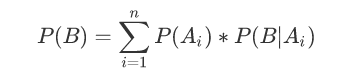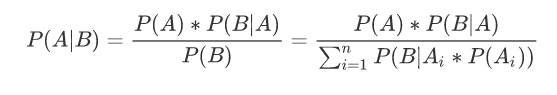### 贝叶斯网络

P(a,b, c) = P(c | a,b)P(b | a)P(a)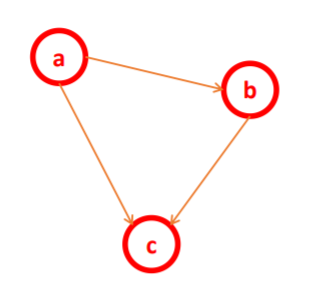### EM算法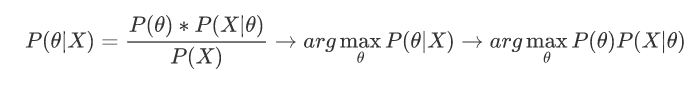EM算法(Expectation Maximization Algorithm, 最大期望算法)是一种迭代类型

EM算法流程：

1. 初始化分布参数

2. 重复下列两个操作直到收敛：

E步骤：估计隐藏变量的概率分布期望函数；

M步骤：根据期望函数重新估计分布参数

EM实现的过程中还会有一个Jensen不等式知识点,它使得我们可以假设隐含数据并形成极大化模型,然后对联合概率求最大值直到收敛。

"""

"""
import numpy as np
from scipy.stats import multivariate_normal
def train(x, max_iter=100):
"""
进行GMM模型训练，并返回对应的μ和σ的值(假定x数据中的簇类别数目为2)
:param x: 输入的特征矩阵x
:param max_iter:  最大的迭代次数
:return:  返回一个五元组(pi, μ1， μ2，σ1，σ2)
"""
# 1. 获取样本的数量m以及特征维度n
m, n = np.shape(x)
# 2. 初始化相关变量
# 以每一列中的最小值作为mu1，mu1中的元素数目就是列的数目(n)个
mu1 = x.min(axis=0)
mu2 = x.max(axis=0)
sigma1 = np.identity(n)
sigma2 = np.identity(n)
pi = 0.5
# 3. 实现EM算法
for i in range(max_iter):
# a. 初始化多元高斯分布（初始化两个多元高斯混合概率密度函数）
norm1 = multivariate_normal(mu1, sigma1)
norm2 = multivariate_normal(mu2, sigma2)
​
# E step
# 计算所有样本数据在norm1和norm2中的概率
tau1 = pi * norm1.pdf(x)
tau2 = (1 - pi) * norm2.pdf(x)
# 概率做一个归一化操作
w = tau1 / (tau1 + tau2)
​
# M step
mu1 = np.dot(w, x) / np.sum(w)
mu2 = np.dot(1 - w, x) / np.sum(1 - w)
sigma1 = np.dot(w * (x - mu1).T, (x - mu1)) / np.sum(w)
sigma2 = np.dot((1 - w) * (x - mu2).T, (x - mu2)) / np.sum(1 - w)
pi = np.sum(w) / m
​
# 返回最终解
return (pi, mu1, mu2, sigma1, sigma2)
​
​
if __name__ == '__main__':
np.random.seed(28)
​
# 产生一个服从多元高斯分布的数据（标准正态分布的多元高斯数据）
mean1 = (0, 0, 0)  # x1\x2\x3的数据分布都是服从正态分布的，同时均值均为0
cov1 = np.diag((1, 1, 1))
data1 = np.random.multivariate_normal(mean=mean1, cov=cov1, size=500)
​
# 产生一个数据分布不均衡
mean2 = (2, 2, 3)
cov2 = np.array([[1, 1, 3], [1, 2, 1], [0, 0, 1]])
data2 = np.random.multivariate_normal(mean=mean2, cov=cov2, size=200)
​
# 合并两个数据
data = np.vstack((data1, data2))
​
pi, mu1, mu2, sigma1, sigma2 = train(data, 100)
print("第一个类别的相关参数:")
print(mu1)
print(sigma1)
print("第二个类别的相关参数:")
print(mu2)
print(sigma2)
​
print("预测样本属于那个类别(概率越大就是那个类别)：")
norm1 = multivariate_normal(mu1, sigma1)
norm2 = multivariate_normal(mu2, sigma2)
x = np.array([0, 1, 0])
print(pi * norm1.pdf(x)) # 属于类别1的概率为:0.0275  => 0.989
print((1 - pi) * norm2.pdf(x))# 属于类别1的概率为:0.0003 => 0.011
​


## HMM详解### HMM介绍

HMM是统计模型，它用来描述一个含有隐含未知参数的马尔可夫过程。其难点是从可观察的参数中确定该过程的隐含参数。然后利用这些参数来作进一步的分析，例如模式识别

### 马尔可夫链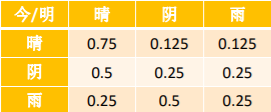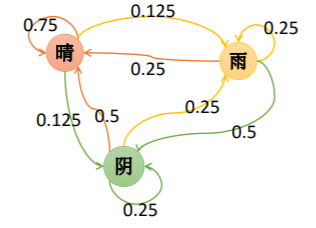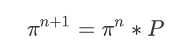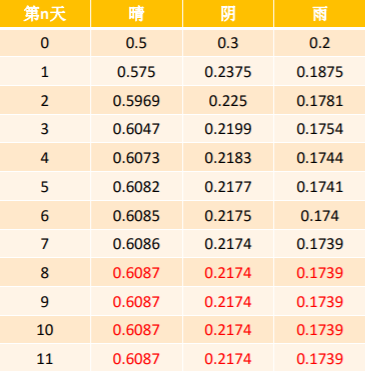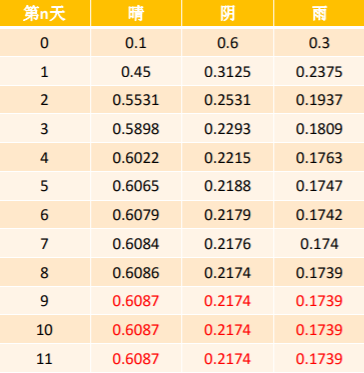### 隐马尔科夫模型

HMM是一种统计模型，在语音识别、行为识别、NLP、故障诊断等领域具有高效的性能。

HMM是关于时序的概率模型，描述一个含有未知参数的马尔可夫链所生成的不可观测的状态随机序列，再由各个状态生成观测随机序列的过程。

HMM是一个双重随机过程---具有一定状态的隐马尔可夫链和随机的观测序列。HMM随机生成的状态随机序列被称为状态序列；每个状态成一个观测，由此产生的观测随机序列，被称为观测序列。

HMM由隐含状态S、可观测状态O、初始状态概率矩阵π、隐含状态转移概率矩阵A、可观测值转移矩阵B(又称为混淆矩阵，Confusion Matrix)；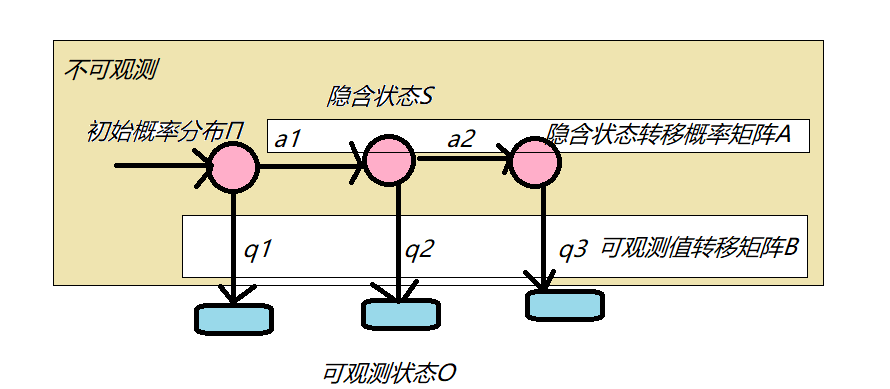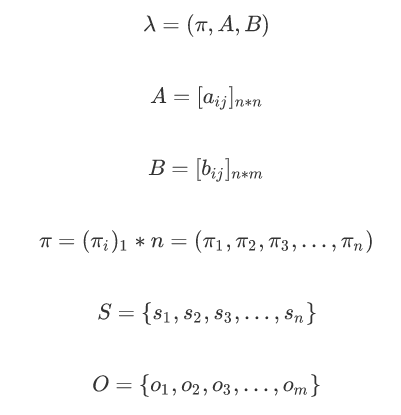这里S和O是相对于整个集合而言的，我们一般只会度量前T个状态序列I和观测序列Q: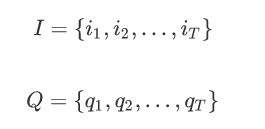### HMM案例

1 4 6
2 8 2
3 5 5

1. 按照π的概率选择一个盒子，从盒子中随机抽取出一个小球(B)，记录颜色后，放回盒子中；

2. 按照某种条件概率(A)选择新的盒子，重复该操作；

3. 最终得到观测序列：“白黑白白黑“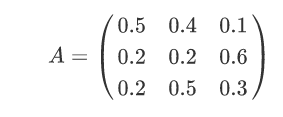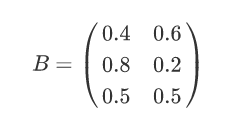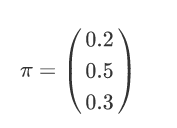1，如何求得一个估计模型，让观测序列在该模型下发生概率最大。

2，如何求得隐藏得状态序列，让它在最优估计模型下发生得概率最大。

## 概率计算问题（前后向）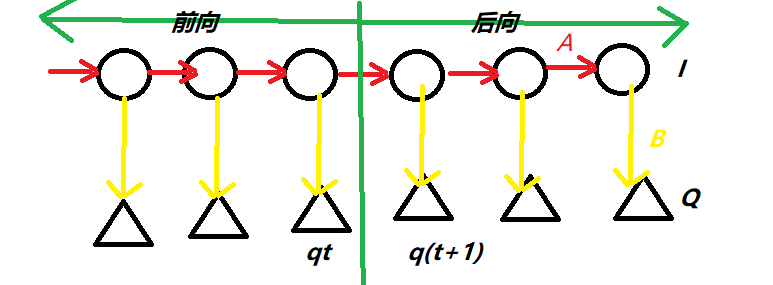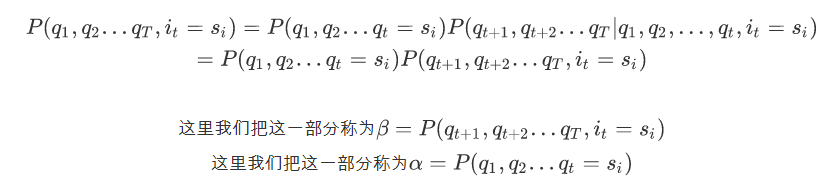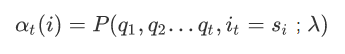1. 初值

先计算第一个α：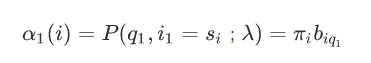2. 递推

求出第一个后,递推使t = 1,2,...,T-1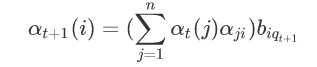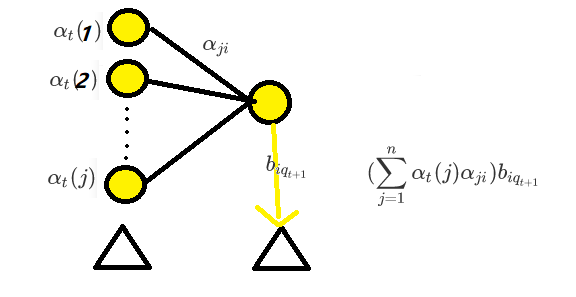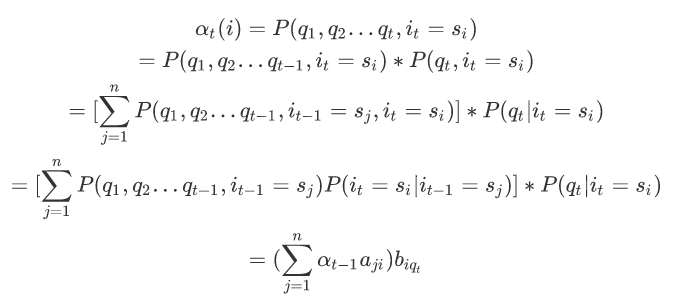3. 结果

这样状态序列为s_i的情况下，观测序列出现的概率就变成了

4.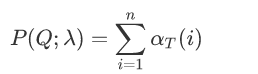5. [前向传播代码]  https://github.com/TimVerion/HMM_code/blob/master/hmm/forward_probability.py 前向传播代码

6. # 伪代码，不可运行
# 更新初值(t=1)
for i in n_range:
alpha[i] = pi[i] * B[i][fetch_index_by_obs_seq_f(Q, 0)]
# 迭代更新其它时刻
T = len(Q)
tmp = [0 for i in n_range]
for t in range(1, T):
for i in n_range:
# 1. 计算上一个时刻t-1累积过来的概率值
for j in n_range:
tmp[j] = alpha[t - 1][j] * A[j][i]
# 2. 更新alpha的值
alpha[t][i] = np.sum(tmp) * B[i][fetch_index_by_obs_seq_f(Q, t)]

后向传播定义:给定λ，定义到时刻t状态为si的前提下，从t+1到T部分观测序列为qt+1,qt+2,...,qT的概率为后向概率。记做: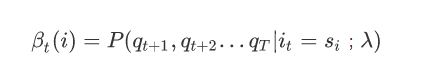​1. 初值

先计算第一个β,在我们进行前向算法的时候我们假设β为1：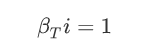7. 递推

求出第一个后,递推使t = T-1,T-2...,1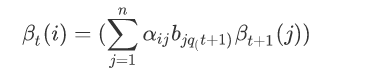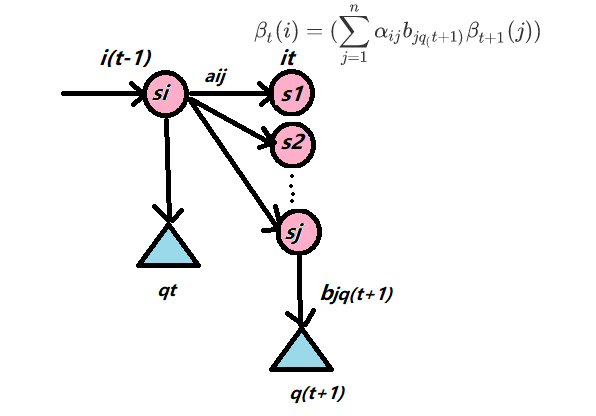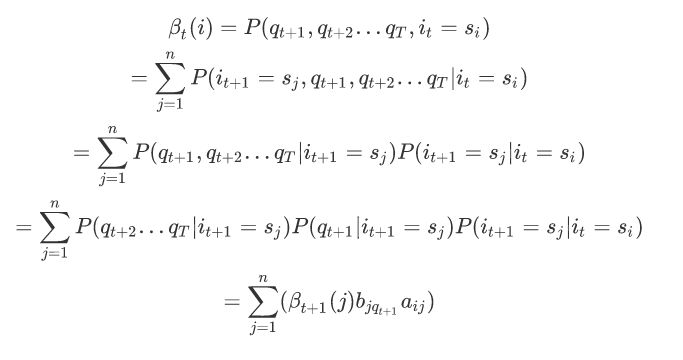8. 结果

这样状态序列为s_i的情况下，观测序列出现的概率就变成了:# 更新初值(t=T)
for i in n_range:
beta[T - 1][i] = 1
# 迭代更新其它时刻
tmp = [0 for i in n_range]
for t in range(T - 2, -1, -1):
for i in n_range:
# 1. 计算到下一个时刻t+1的概率值
for j in n_range:
tmp[j] = A[i][j] * beta[t + 1][j] * B[j][fetch_index_by_obs_seq_f(Q, t +1)]
# 2. 更新beta的值
beta[t][i] = np.sum(tmp)

## 学习问题(鲍姆韦尔奇)

#### 1,单个状态的概率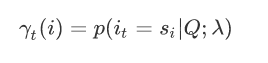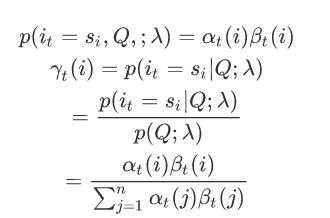#### 2,两个状态的联合概率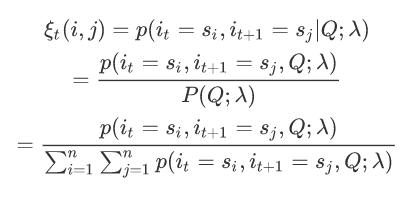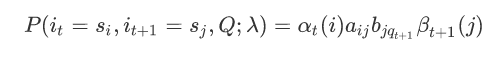### 3,解决学习问题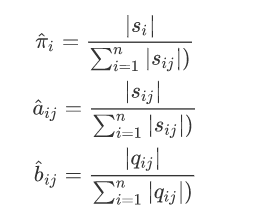1. 初始化分布参数

2. 重复下列两个操作直到收敛：

E步骤：估计隐藏变量的概率分布期望函数: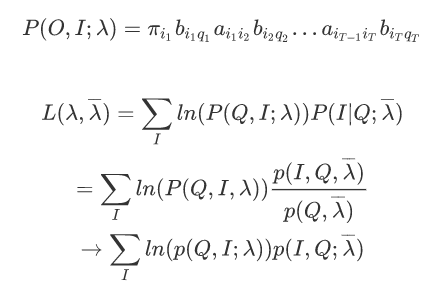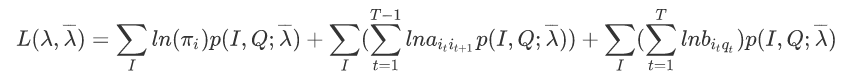### M步骤：根据期望函数重新估计分布参数

##### π的求解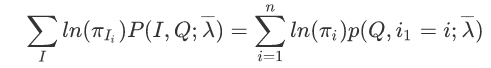##### A的求解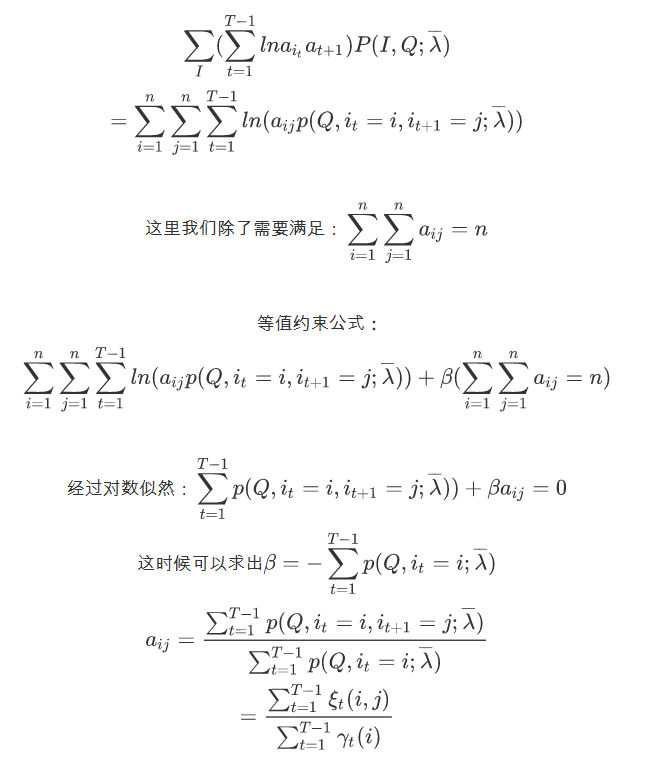#### B的求解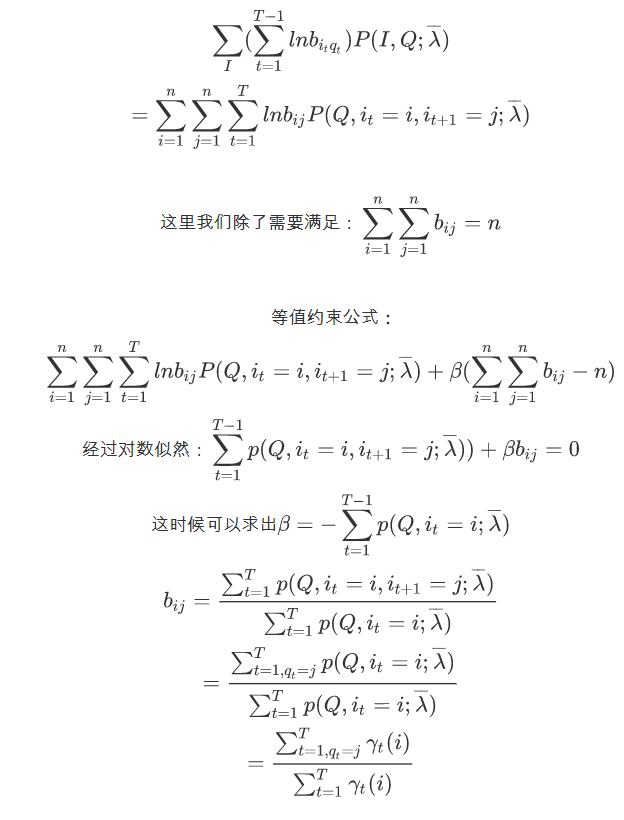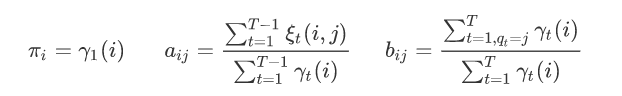# 1. 迭代更新(EM算法思想类型)
for time in range(max_iter):
# a. 在当前的pi，A，B的情况下对观测序列Q分别计算alpha、beta、gamma和ksi
forward.calc_alpha(pi, A, B, Q, alpha, fetch_index_by_obs_seq_f)
backward.calc_beta(pi, A, B, Q, beta, fetch_index_by_obs_seq_f)
single.calc_gamma(alpha, beta, gamma)
continuous.calc_ksi(alpha, beta, A, B, Q, ksi, fetch_index_by_obs_seq
# b. 更新pi、A、B的值
# b.1. 更新pi值
for i in n_range:
pi[i] = gamma
# b.2. 更新状态转移矩阵A的值
tmp1 = np.zeros(T - 1)
tmp2 = np.zeros(T - 1)
for i in n_range:
for j in n_range:
# 获取所有时刻从状态i转移到状态j的值
for t in t_1_range:
tmp1[t] = ksi[t][i][j]
tmp2[t] = gamma[t]
# 更新状态i到状态j的转移概率
A[i][j] = np.sum(tmp1) / np.sum(tm
# b.3. 更新状态和观测值之间的转移矩阵
for i in n_range:
for k in m_range:
tmp1 = np.zeros(T)
tmp2 = np.zeros(T)
# 获取所有时刻从状态i转移到观测值k的概率和
number = 0
for t in t_range:
if k == fetch_index_by_obs_seq_f(Q, t):
如果序列Q中时刻t对应的观测值就是k，那么进行统计这个时刻t为状态i的概率值
tmp1[t] = gamma[t][i]
number +
tmp2[t] = gamma[t]
# 更新状态i到观测值k之间的转移概率
if number == 0:
# 没有转移，所以为0
B[i][k] = 0
else:
# 有具体值，那么进行更新操作
B[i][k] = np.sum(tmp1) / np.sum(tem2)

## 预测问题（维特比）

Viterbi算法实际是用动态规划的思路求解HMM预测问题，求出概率最大的“路径”，每条“路径”对应一个状态序列。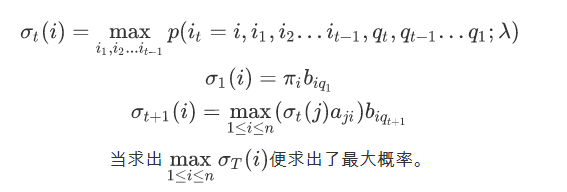# 1. 计算t=1的时候delta的值
for i in n_range:
delta[i] = pi[i] * B[i][fetch_index_by_obs_seq_f(Q, 0)]
# 2. 更新其它时刻的值
for t in range(1, T):
for i in n_range:
# 当前时刻t的状态为i
# a. 获取最大值
max_delta = -1
for j in n_range:
# j表示的是上一个时刻的状态值
tmp = delta[t - 1][j] * A[j][i]
if tmp > max_delta:
max_delta = tmp
pre_index[t][i] = j
# b. 更新值
delta[t][i] = max_delta * B[i][fetch_index_by_obs_seq_f(Q, t)]
# 3. 解码操作，查找到最大的结果值
decode = [-1 for i in range(T)]

HMM在我们生活中无处不在，举个简单的例子：

## 马尔可夫模型解决语音识别

posted @ 2019-07-15 08:10  Timcode  阅读(722)  评论(0编辑  收藏